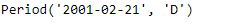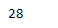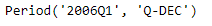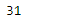# Python | Pandas Period.daysinmonth

• Last Updated : 06 Jan, 2019

Python is a great language for doing data analysis, primarily because of the fantastic ecosystem of data-centric python packages. Pandas is one of those packages and makes importing and analyzing data much easier.

Pandas` Period.daysinmonth` attribute returns the number of days in the month present in the given Period object.

Attention geek! Strengthen your foundations with the Python Programming Foundation Course and learn the basics.

To begin with, your interview preparations Enhance your Data Structures concepts with the Python DS Course. And to begin with your Machine Learning Journey, join the Machine Learning - Basic Level Course

Syntax : Period.daysinmonth

Parameters : None

Return : number of days

Example #1: Use `Period.daysinmonth` attribute to find the number of days present in the month in the given Period object.

 `# importing pandas as pd``import` `pandas as pd`` ` `# Create the Period object``prd ``=` `pd.Period(freq ``=``'D'``, year ``=` `2001``, month ``=` `2``, day ``=` `21``)`` ` `# Print the Period object``print``(prd)`

Output :Now we will use the `Period.daysinmonth` attribute to find the number of days present in the month represented in the given period object.

 `# return the number of days``prd.daysinmonth`

Output :As we can see in the output, the `Period.daysinmonth `attribute has returned 28 indicating that the month represented in the given period object has 28 days.

Example #2: Use `Period.daysinmonth` attribute to find the number of days present in the month in the given Period object.

 `# importing pandas as pd``import` `pandas as pd`` ` `# Create the Period object``prd ``=` `pd.Period(freq ``=``'Q'``, year ``=` `2006``, quarter ``=` `1``)`` ` `# Print the object``print``(prd)`

Output :Now we will use the `Period.daysinmonth` attribute to find the number of days present in the month represented in the given period object.

 `# return the number of days``prd.daysinmonth`

Output :As we can see in the output, the `Period.daysinmonth `attribute has returned 31 indicating that the month represented in the given period object has 31 days.

My Personal Notes arrow_drop_up